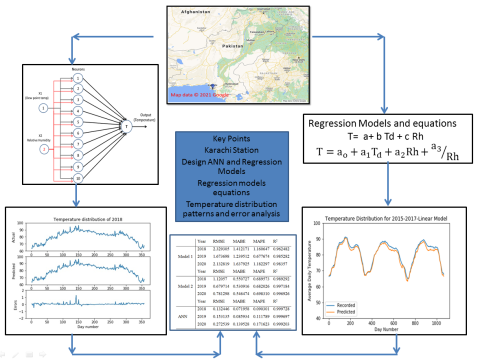# New Regression models for Estimation daily temperature of Karachi and its Neural Network analysis

Paper Topic:
Environmental data analysis and modelling

Volume:

Issue:

Pages :
519 - 525

Corresponing Author:

Authors:
Paper ID:
gnest_03953
Paper Status:
Published
Date Paper Accepted:
29-10-2021
Paper online:
30/10/2021
Visual abstract:Abstract:

This study presents the determination of the average daily temperature distribution for Karachi city. Artificial Neural Network (ANN) has been used to predict the average daily temperature of 2018, 2019, and 2020. Two regression models (linear and non-linear) were also developed. These models are based on relative humidity and dew points. Karachi's six-year environmental datasets were used for the case study location and to establish temperature distribution models. In ANN three years, temperature data (2015-2017) was used to train and validate the NN model. The same data was used to find the regression coefficients of each model. Both models and NN are then used to estimate the average daily temperature of years 2018-2020. The statistical errors are also calculated for comparison and to evaluate the performance of both models; an excellent agreement was found between recorded and ANN estimates. Both regression models predict average daily temperature with reasonable uncertainties. However, the non-linear regression model predictions are better. The results show that the models provide a good prediction of temperature distribution.

Keywords:
Neural network, regression, Karachi, mathematical modeling, temperature distribution